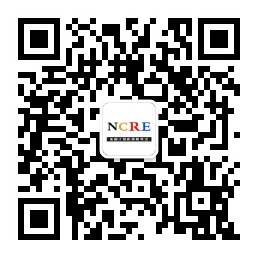400-8077-735

# NCRE等级考试《二级 月 C语言》考试题库一

1.下列叙述中正确的是（ ）。

A. 所有数据结构必须有根结点

B. 所有数据结构必须有终端结点(即叶子结点)

C. 只有一个根结点，且只有一个叶子结点的数据结构一定是线性结构

D. 没有根结点或没有叶子结点的数据结构一定是非线性结构

【答案】:D

【解析】:

D项正确，线性结构的特点是：①集合中必存在“第一个元素”且惟一；②集合中必存在“最后一个元素”且惟一；③除最后一个元素外，其他数据元素均有惟一的“后继”；④除第一个元素外，其他数据元素均有惟一的“前驱”。所以没有根结点或没有叶子结点的数据结构一定是非线性结构。AB两项错误，不是所有数据结构都必须有根结点和叶子结点；C项错误，数据结构中若有中间结点不满足只有一个前件或者后件的条件，就不是线性结构。答案选择D选项。

2.以下叙述中错误的是（ ）。

A. C语言中的每条可执行语句和非执行语句最终都将被转换成二进制的机器指令

B. C程序经过编译、链接步骤之后才能形成一个真正可执行的二进制机器指令文件

C. 用C语言编写的程序称为源程序，它以ASCII代码形式存放在一个文本文件中

D. C语言源程序经编译后生成后缀为.obj的目标程序

【答案】:A

【解析】:

3.C语言主要是借助以下（ ）功能来实现程序模块化的。

A. 定义函数

B. 定义常量和外部变量

C. 三种基本结构语句

D. 丰富的数据类型

【答案】:A

【解析】:

C程序的模块化主要通过函数来实现。C语言允许对函数单独进行编译，从而可以实现模块化。答案选择A选项。

4.有以下程序段：

charch;

int k;

ch='a';

k =12;

printf("%c,%d,",ch,ch,k);

printf("k=%d\n",k);

A. 因变量类型与格式描述符的类型不匹配输出无定值

B. 输出项与格式描述符个数不符，输出为零值或不定值

C.a,97,12k=12

D.a,97,k=12

【答案】:D

【解析】:

5.下列叙述中正确的是（ ）。

A. 栈是“先进先出”的线性表

B. 队列是“先进后出”的线性表

C. 循环队列是非线性结构

D. 有序线性表既可以采用顺序存储结构，也可以采用链式存储结构

【答案】:D

【解析】:

6.若要实现total=1+2+3+4+5求和，以下程序段错误的是（ ）。

A.

int i=1,total=1;

while(i<5)

{

total+=i;

i+=1;

}

B.

int i=1,total=0;

while(i<=5)

{

total+=i;

i+=1;

}

C.

int i=0,total=0;

while(i<5)

{

i+=1;

total+=i;

}

D.

int i=0,total=0;

while(i<=5)

{

total+=i;

i+=1;

}

【答案】:A

【解析】:

A项执行过程为：i=1，total=1，判断while循环条件1<5成立，total=1+1，i=2；判断循环条件执行函数体，直到i=4，total=1+1+2+3+4，i=5；i=5循环条件不成立，退出循环，A项不能实现题目要求，错误。B项执行过程：i=1，total=0，1<=5成立，total=0+1=1，i=2；直到i=5，total=1+2+3+4+5，i=6；i=6条件不成立，退出循环，程序正确。C项执行过程：i=0，total=0，0<5成立，i=1，total=1；直到i=5，total=1+2+3+4+5；i=5条件不成立，退出循环，程序正确。D项执行过程：i=0，total=0，0<=5成立，total=0，i=1；直到i=5，total=1+2+3+4+5，i=6；i=6条件不成立，退出循环，程序正确。答案选择A选项。

7.下列不属于软件工程3个要素的是（ ）。

A. 工具

B. 过程

C. 方法

D. 环境

【答案】:D

【解析】:

8.下面不属于黑盒测试方法的是（ ）。

A. 边界值分析法

B. 基本路径测试

C. 等价类划分法

D. 错误推测法

【答案】:B

【解析】:《计算机等级考试网》免责声明：

1、因考试政策、内容不断变化与调整，本网站提供的以上信息仅供参考，如有异议，请考生以权威部门公布的内容为准！

2、本网信息来源为其他媒体的稿件转载，免费转载出于非商业性学习目的，版权归原作者所有，如有内容与版权问题等请与本站联系。联系邮箱：812379481@qq.com。

### 互动交流

• ###### 扫码进入微信刷题助手解锁即可开始刷题

并加入考生交流群

• ###### 扫码加入微信交流群与广大计算机等级考生

共同学习交流